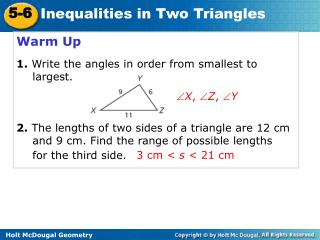Download PresentationWarm Up 1. Write the angles in order from smallest to largest.

# Warm Up 1. Write the angles in order from smallest to largest. - PowerPoint PPT Presentation

Warm Up 1. Write the angles in order from smallest to largest. 2. The lengths of two sides of a triangle are 12 cm and 9 cm. Find the range of possible lengths for the third side.  X ,  Z ,  Y. 3 cm < s < 21 cm. 5-6. Inequalities in Two Triangles. Warm Up. Lesson Presentation.I am the owner, or an agent authorized to act on behalf of the owner, of the copyrighted work described.
Download Presentation## Warm Up 1. Write the angles in order from smallest to largest.

Download Policy: Content on the Website is provided to you AS IS for your information and personal use and may not be sold / licensed / shared on other websites without getting consent from its author.While downloading, if for some reason you are not able to download a presentation, the publisher may have deleted the file from their server.

- - - - - - - - - - - - - - - - - - - - - - - - - - E N D - - - - - - - - - - - - - - - - - - - - - - - - - -
Presentation Transcript
1. Warm Up 1.Write the angles in order from smallest to largest. 2. The lengths of two sides of a triangle are 12 cm and 9 cm. Find the range of possible lengths for the third side. X, Z, Y 3 cm < s < 21 cm

2. 5-6 Inequalities in Two Triangles Warm Up Lesson Presentation Lesson Quiz Holt McDougal Geometry Holt Geometry

3. Objective Apply inequalities in two triangles.

4. Example 1A: Using the Hinge Theorem and Its Converse Compare mBACand mDAC. Compare the side lengths in ∆ABC and ∆ADC. AB = AD AC = AC BC > DC By the Converse of the Hinge Theorem, mBAC > mDAC.

5. Example 1B: Using the Hinge Theorem and Its Converse Compare EF and FG. Compare the sides and angles in ∆EFH angles in ∆GFH. mGHF = 180° – 82° = 98° EH = GH FH = FH mEHF > mGHF By the Hinge Theorem, EF < GF.

6. Example 1C: Using the Hinge Theorem and Its Converse Find the range of values for k. Step 1 Compare the side lengths in ∆MLN and ∆PLN. LN = LN LM = LP MN > PN By the Converse of the Hinge Theorem, mMLN > mPLN. 5k – 12 < 38 Substitute the given values. k < 10 Add 12 to both sides and divide by 5.

7. Example 1C Continued Step 2 Since PLN is in a triangle, mPLN > 0°. 5k – 12 > 0 Substitute the given values. k < 2.4 Add 12 to both sides and divide by 5. Step 3 Combine the two inequalities. The range of values for k is 2.4 < k < 10.

8. Check It Out! Example 1a Compare mEGHand mEGF. Compare the side lengths in ∆EGH and ∆EGF. FG = HGEG = EGEF > EH By the Converse of the Hinge Theorem, mEGH< mEGF.

9. Check It Out! Example 1b Compare BC and AB. Compare the side lengths in ∆ABD and ∆CBD. AD = DC BD = BD mADB > mBDC. By the Hinge Theorem, BC > AB.

10. Example 2: Travel Application John and Luke leave school at the same time. John rides his bike 3 blocks west and then 4 blocks north. Luke rides 4 blocks east and then 3 blocks at a bearing of N 10º E. Who is farther from school? Explain.

11. Example 2 Continued The distances of 3 blocks and 4 blocks are the same in both triangles. The angle formed by John’s route (90º) is smaller than the angle formed by Luke’s route (100º). So Luke is farther from school than John by the Hinge Theorem.

12. Check It Out! Example 2 When the swing ride is at full speed, the chairs are farthest from the base of the swing tower. What can you conclude about the angles of the swings at full speed versus low speed? Explain. The  of the swing at full speed is greater than the  at low speed because the length of the triangle on the opposite side is the greatest at full swing.

13. Example 3: Proving Triangle Relationships Write a two-column proof. Given: Prove: AD > CB Proof: 1. Given 2. Reflex. Prop. of  3. Hinge Thm.

14. Check It Out! Example 3a Write a two-column proof. Given: C is the midpoint of BD. m1 = m2 m3 > m4 Prove: AB > ED

15. Proof: 1. Given 1.C is the mdpt. of BD m3 > m4, m1 = m2 2. Def. of Midpoint 3.1  2 3. Def. of  s 4. Conv. of Isoc. ∆ Thm. 5.AB > ED 5. Hinge Thm.

16. Check It Out! Example 3b Write a two-column proof. Given: SRT  STR TU > RU Prove: mTSU > mRSU 1. Given 1.SRT  STR TU > RU 2. Conv. of Isoc. Δ Thm. 3. Reflex. Prop. of  4. mTSU > mRSU 4. Conv. of Hinge Thm.

17. Lesson Quiz: Part I 1. Compare mABCand mDEF. 2. Compare PS and QR. mABC> mDEF PS < QR

18. Lesson Quiz: Part II 3. Find the range of values for z. –3 < z < 7

19. 1. Given 2. Reflex. Prop. of  3. Conv. of Hinge Thm. 3. mXYW <mZWY Lesson Quiz: Part III 4. Write a two-column proof. Prove: mXYW <mZWY Given: Proof: